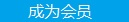您可以捐助，支持我们的公益事业。 1元 10元 50元 认证码：必填求知 文章 文库 Lib 视频 Code iProcess 课程 角色 咨询 工具 火云堂 讲座吧 建模者要资料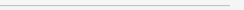订阅捐助
.net中的泛型全面解析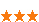489 次浏览     评价： 好 中 差

1，类型安全性

2，性能提高

3，代码重用

4，扩展性

 public class Person { private T _t; public T T1 { get { return _t; } set { _t = value; } } private string _firstName; public string FirstName { get { return _firstName; } set { _firstName = value; } } private string _lastName; public string LastName { get { return _lastName; } set { _lastName = value; } } public Person() { } public Person(T t1, string firstName, string lastName) { this._t = t1; this._firstName = firstName; this._lastName = lastName; } }

 Person person = new Person(1, "Edrick", "Liu"); Person personString = new Person ("ET001", "Edrick", "Liu"); Console.WriteLine("INT:ID:{0},FirstName:{1}, LastName:{2}",person.T1,person.FirstName, person.LastName); Console.WriteLine("STRING:ID:{0},FirstName: {1},LastName:{2}",personString.T1,personString. FirstName,personString.LastName);

 public class MyID { private string _city; public string City { get { return _city; } set { _city = value; } } private string _school; public string School { get { return _school; } set { _school = value; } } private string _className; public string ClassName { get { return _className; } set { _className = value; } } private string _number; public string Number { get { return _number; } set { _number = value; } } public MyID() { } public MyID(string city, string school, string className, string number) { this._city = city; this._school = school; this._className = className; this._number = number; } }

 MyID myId =new MyID("WuHan", "SanZhong", "YiBan", "0001"); Person personID = new Person(myId, "Edrick", "Liu"); Console.WriteLine("ID:{0},FirstName:{1},LastName:{2}",myId.City+ "-"+myId.School+"-"+myId.ClassName+"-"+myId.Number,personID. FirstName,personID.LastName);

 ArrayList list = new ArrayList(); list.Add(1); list.Add(2); list.Add(3); IEnumerator ie = list.GetEnumerator(); while (ie.MoveNext()) { int i = (int)ie.Current; Console.WriteLine(i); }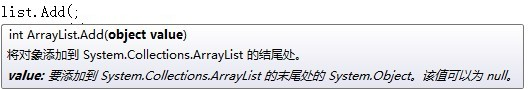ArrayList list = new ArrayList(); list.Add(1); list.Add(2); list.Add(3); list.Add("e");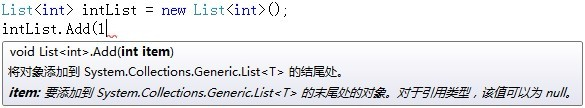public class PerosnMoreTypeOfT { private TId _id; public TId Id { get { return _id; } set { _id = value; } } private TFirstName _firstName; public TFirstName FirstName { get { return _firstName; } set { _firstName = value; } } private TLastName _lastName; public TLastName LastName { get { return _lastName; } set { _lastName = value; } } public PerosnMoreTypeOfT() { } public PerosnMoreTypeOfT(TId tId, TFirstName tFirstName, TLastName tLastName) { this._id = tId; this._firstName = tFirstName; this._lastName = tLastName; } } 　　调用代码 PerosnMoreTypeOfT person = new PerosnMoreTypeOfT (1, "Edrick", "Liu"); Console.WriteLine("ID:{0},FirstName:{1},LastName: {2}",person.Id,person.FirstName,person.LastName);

where T : struct -类型T必须是值类型

where T : class -类型T必须是引用类型

where T : Ifoo -类型T必须执行接口Ifoo

where T : foo -类型T必须继承自 foo

where T : new() -类型T必须有一个默认构造函数

where T : U -指定泛型类型T1派生于T2。

 public class Personwhere T:struct

 public interface IPerson { void DisplayPerosnWithOutId(); void DisplayPerosnWithId(); } 　　定义接口。 public class Person:IPerson where T:MyID { private T _t; public T T1 { get { return _t; } set { _t = value; } } private string _firstName; public string FirstName { get { return _firstName; } set { _firstName = value; } } private string _lastName; public string LastName { get { return _lastName; } set { _lastName = value; } } public Person() { } public Person(T t1, string firstName, string lastName) { this._t = t1; this._firstName = firstName; this._lastName = lastName; } public void DisplayPerosnWithOutId() { Console.WriteLine("FirstName:{0},LastName :{1}",FirstName,LastName); } public void DisplayPerosnWithId() { MyID myId = T1; Console.WriteLine("ID:{0},FirstName:{1} ,LastName:{2}", myId.City + "-" + myId. School + "-" + myId.ClassName + "-" + myId.Number, FirstName, LastName); } }

 public class DisPersonwhere T:IPerson { private T t { get; set; } public DisPerson() { } public DisPerson(T t1) { this.t = t1; } public void dis() { IPerson p = (IPerson)t; p.DisplayPerosnWithId(); p.DisplayPerosnWithOutId(); } }

 MyID myId = new MyID("WuHan", "SanZhong", "YiBan", "0001"); Person perosn = new Person (myId,"Edrick","Liu"); DisPerson> dis = new DisPerson>(perosn); dis.dis();

 public class ClassA { } public class ClassB:ClassA { } public class ClassC where TClassB:TClassA { }

 ClassA a = new ClassA(); ClassB b = new ClassB(); ClassC c = new Class C();

Default关键字

default关键字其实不需要解释太多，这里只解释一下原理就行了。我们前面提到，泛型只是一个模板类型，就是我们在定义的时候根本就不可能知道用户在实例化的时候会以何种类型来替换。有可以是值类型，也有可能是引用类型。值类型是不能赋值为null的。所以泛型类型不能赋值为null，但是这里仍然有50%的几率是引用类型，我们还是需要50%的机会需要泛型T为null。这时就需要default关键字。

 private T t =default(T);

 StaticGeneric.x = 5; StaticGeneric.x = 7; StaticGeneric.x = 6; Console.WriteLine(StaticGeneric.x); Console.WriteLine(StaticGeneric.x);

 StaticGeneric.x = 5; StaticGeneric.x = 7; StaticGeneric.x = 6; Console.WriteLine(StaticGeneric.x); Console.WriteLine(StaticGeneric.x);

 public abstract class Basewhere T:struct { private int _id; public int Id { get { return _id; } set { _id = value; } } public abstract T Add(T x, T y); }

 public class SonClass:Base { private T _t; public T T1 { get { return _t; } set { _t=value;} } public SonClass() { } public SonClass(T t,int id) { this._t = t; base.Id = id; } public override int Add(int x, int y) { return x + y; } public void Prit() { Console.WriteLine(T1); } }

 SonClass son = new SonClass ("EdrickLiu",10); Console.WriteLine(son.Id); Console.WriteLine(son.Add(3,5)); son.Prit();

 public interface ICompare where T:class { bool CompareTo(T one,T other); }

 public class CompareClass:ICompare { public bool CompareTo(MyID one, MyID other) { if (one != null && other != null) { if (one.City == other.City && one. School == other.School & one.ClassName == other.ClassName && one.Number == other.Number) { return true; } else { return false; } } else { return false; } } }

 MyID id = new MyID("Wuhan", "Shanzhong", "YiBan", "ET001"); MyID myid = new MyID("Wuhan", "Shanzhong", "YiBan", "ET002"); ICompare compare = new CompareClass(); Console.WriteLine(compare.CompareTo(id, myid));

 public static void Swap(ref T x,ref T y) { T temp; temp = x; x = y; y = temp; }

 int x = 8; int y = 9; GenericMethod.Swap(ref x,ref y); Console.WriteLine("X:{0},Y:{1}",x,y);

 public class SalaryPerson { private int _id; public int Id { get { return _id; } set { _id = value; } } private string _name; public string Name { get { return _name; } set { _name = value; } } private decimal _salary; public decimal Salary { get { return _salary; } set { _salary = value; } } public SalaryPerson() { } public SalaryPerson(int id, string name, decimal salry) { this._id = id; this._name = name; this._salary = salry; } }

 public static decimal AddSalary (IEnumerable e) { decimal total = 0; foreach (SalaryPerson p in e) { total += p.Salary; } return total; }

 public static decimal AddSalary (IEnumerable e) where T:SalaryPerson { decimal total = 0; foreach (T t in e) { total += t.Salary; } return total; }

 public delegate TResult Action(TInput input,TResult result); public static TResult GetSalary(IEnumerable e, Action action) { TResult result =default(TResult); foreach (TInput t in e) { result = action(t,result); } return result; } }

 List list = new List (); list.Add(new SalaryPerson(1,"Edrick",5000)); list.Add(new SalaryPerson(1,"Meci",3000)); list.Add(new SalaryPerson(1,"Jun",1000)); GenericAndDelegate.Action a = new GenericAndDelegate.Action (GetS); decimal d = GenericAndDelegate.GetSalary (list, a); Console.WriteLine(d); Console.Read(); } public static decimal GetS(SalaryPerson p,decimal d) { d += p.Salary; return d; }

 GenericAndDelegate.Action a = GetS;

 decimal d = GenericAndDelegate.GetSalary(list, GetS);

 GenericAndDelegate.Action a = delegate(SalaryPerson p, decimal d1) { d1 += p.Salary; return d1; }; decimal d = GenericAndDelegate.GetSalary (list, a);

 decimal d = GenericAndDelegate.GetSalary (list, (p,dl)=>dl+ =p.Salary);

 decimal d = GenericAndDelegate.GetSalary (list, (SalaryPerson p,decimal dl)=>dl+=p.Salary);

 decimal d = GenericAndDelegate.GetSalary SalaryPerson, Decimal>(list, (SalaryPerson p,decimal dl)=>dl+=p.Salary/10);

 int[] ints = { 2,4,6,5,8,9,10}; Array.Sort(ints, (i, j) => i.CompareTo(j)); Array.ForEach(ints, i => Console.WriteLine(i*2)); var query = ints.Where(i => i > 2); foreach (int i in query) { Console.WriteLine(i); } Console.Read();

List<int>和List<int>会是同个版本不同实例，但是他们共享list<int>的单个实例。

List<int>和List<long>会创造不同的版本，不同的实例。这就是我们上面说的静态值不同的原因。

List<string>和List<object>或创造同一版本但是实例不同。

 List

 public void Convert(IList input,IList outo) where TInput:TOut { foreach (TInput t in input) { outo.Add(t); } }

 int[] ints = { 2,3,4,5,6,7,8,9}; ArraySegment arr = new ArraySegment (ints, 2, 3); for (int i = arr.Offset; i < arr.Count+ arr.Offset; i++) { Console.WriteLine(arr.Array[i]); }

Offset是相对原数组的索引，而count是现在的容量489 次浏览  评价: 好 中 差订阅捐助
 相关文章 深度解析：清理烂代码 如何编写出拥抱变化的代码 重构-使代码更简洁优美 团队项目开发"编码规范"系列文章
 相关文档 重构-改善既有代码的设计 软件重构v2 代码整洁之道 高质量编程规范
 相关课程 基于HTML5客户端、Web端的应用开发 HTML 5+CSS 开发 嵌入式C高质量编程 C++高级编程每天2个文档/视频 扫描微信二维码订阅
 订阅技术月刊 获得每月300个技术资源